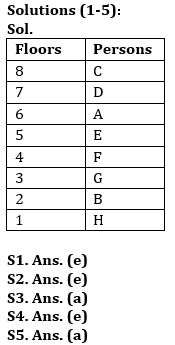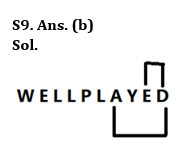Latest Banking jobs   »

# Reasoning Quiz For Bank of Baroda SO 2023 -26th May

Directions (1-5): Study the information carefully and answer the question given below.
There are eight persons A, B, C, D, E, F, G and H live in an eight-floor building (Ground floor is numbered 1 and topmost floor is number 8.) but not necessary in the same order.
F lives on floor number 4. G lives on an odd number floor below 6th floor. D lives on one of the odd number floors but not below G’s floor. There is one person living between C and A who lives just above E. B lives on the floor immediate above H. C lives on a floor above A. Not more than two persons live between A and G.

Q1. On which of the following floor G lives?
(a) Either 3rd or 1st
(b) 5th
(c) 7th
(d) 1st
(e) 3rd

Q2. How many persons live between D and C?
(a) One
(b) Two
(c) Three
(d) Four
(e) None

Q3. If all the persons are arranged alphabetically from top to bottom then how many persons remain (live) on same floor?
(a) One
(b) Two
(c) Three
(d) Four
(e) None

Q4. Four of the following five are alike in a certain way based on their arrangement, find the one who does not belong to that group?
(a) B
(b) A
(c) F
(d) C
(e) G

Q5. If C is related to A, in the same way D is related to E, then following the same pattern, F is related to ___?
(a) B
(b) A
(c) E
(d) C
(e) None of these

Q6. If it is possible to make a meaningful word from 1st, 3rd, 4th, 5th and 6th letters of word “SPELLCHECKER”, then which will be the 2nd letter of the word (from left)? If no such word be formed, mark the answer as ‘Y’. If more than one word can be formed mark the answer as ‘X’.
(a) Y
(b) L
(c) E
(d) C
(e) X

Q7. If it is possible to make a meaningful word from 1st, 2nd, 5th, and 6th letters of word “PARTIALLY”, then which will be the 3rd letter of the word? If no such word be formed, mark the answer as ‘Y’. If more than one word can be formed mark the answer as ‘X’.
(a) Y
(b) P
(c) A
(d) T
(e) X

Q8. How many pairs of letters are in the word “ANAGRAMS” which has as many letters between them as in the alphabetical series (Both forward and backward direction)?
(a) One
(b) Two
(c) Three
(d) Four
(e) None

Q9. How many pairs of letters are in the word “WELLPLAYED” which has as many letters between them as in the alphabetical series (Both forward and backward direction)?
(a) One
(b) Two
(c) Three
(d) Four
(e) None

Q10. If all the even digits of the number “547658693” are decreased by ‘1’ and then all the digits are arranged in ascending order from left to right, then what will be the difference between fourth digit from left end and second digit from the right end?
(a) 3
(b) 4
(c) 6
(d) 2
(d) None of these

SolutionsS6. Ans. (c)
Sol. 1st, 3rd, 4th, 5th and 6th letters of word – S, E, L, L, C
So, meaningful word – CELLS. Hence, E is the correct answer.

S7. Ans. (a)
Sol. 1st, 2nd, 5th, and 6th letters of word – P, A, I, A
Hence, no meaningful word can be formed. Hence, Y is the correct answer.

S8. Ans. (e)
Sol. There is no such pair.S10. Ans. (d)
Sol. Given Number – 547658693
After operation – 537557593
ascending Order – 335555779
Required difference = 7 – 5 = 2.## FAQs

### What is the selection process of Bank of Baroda AO 2023?

The selection process of Bank of Baroda AO consists of Online exam and Interview

#### Congratulations!Union Budget 2023-24: Free PDF